: Linear Josephus Problem. : The Self-Similarity of the Josephus Problems. : Introduction and the traditional Josephus Problem.

# The Josephus Problem in both Directions.

Here we are going to introduce the Josephus Problem in both directions. This variant of the Josephus Problem has been introduced by the authors in .

Definition 2.1   In this variant of the Josephus Problem two numbers are to be removed at the same time, but two processes of elimination go for different directions. Letandbe natural numbers such that. Suppose that there are-numbers. Then the first process of elimination starts counting up from the first number and remove the-th,-th,-th number, ... . The second process counts down from the-th number and remove the-th,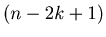-th,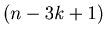-th number, ... . We suppose that the first process comes first and the second process second at every stage. We denote the position of the survivor by.

The authors studied this varinat of Josephus problem for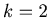, and discovered some very interesting self-similarity in the problem. See 

Example 2.1   This is a Mathematica program to calculate. The following Mathematica function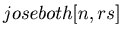returns the value of.
```joseboth[m_, mm_] := Block[{t, p, q, u, v, w}, w = mm - 1;
t = Range[m];
p = t;
q = t;
Do[p = RotateLeft[p, w];
u = First[p];
p = Rest[p];
q = Drop[q, Position[q, u][]];
If[Length[p] == 1, Break[],];
q = RotateRight[q, w];
v = Last[q];
q = Drop[q, -1];
p = Drop[p, Position[p, v][]];
If[Length[q] == 1, Break[],], {n, 1, Ceiling[m/2]}]; p[]];
```

By using the Mathematica function in Example 2.1 we can make the list { JI(n,k), n = 1,2,... }.

Example 2.2   Graph 2.1 and Graph 2.2 are the graphs of the lists {, n = 1,2,..,1024} and {, n = 1,2,..,256}.
The horizontal coordinate is for the number of numbers in the game, and the vertical coordinate is for the number that remains.
Please compare these two graphs．They have the same shape, and hence these graphs seem to have Fractal behavior (self-similarity). Note that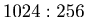=. This can be seen as the ratio of the self-similarity of the graph.

Graph 2.1Graph 2.2The authors could prove that the graph ofin Example 2.2 has the self-similarity with the ratio ofin . The authors have not proved the existence of the self-similarity for. As to these problems the authors are going to present only graphs, and the graphs seem to have the self-similarity.

Example 2.3   Graph 2.3 and Graph 2.4 are the graphs of the lists {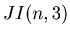, n = 1,2,..,1024} and {, n = 1,2,..,455}. Please compare these two graphs．They have the same shape, and hence these graphs seem to have Fractal behavior (self-similarity). Note thatis approximately equal to.

Graph 2.3Graph 2.4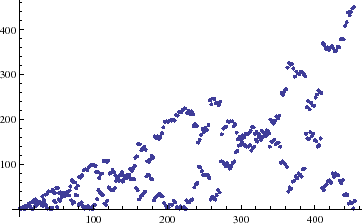Example 2.4   Graph 2.5 and Graph 2.6 are the graphs of the lists {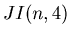, n = 1,2,..,1024} and {, n = 1,2,..,580}. Please compare these two graphs．They have the same shape, and hence these graphs seem to have Fractal behavior (self-similarity). Note thatis approximately equal to.

Graph 2.5Graph 2.6Example 2.5   Graph 2.7 and Graph 2.8 are the graphs of the lists {, n = 1,2,..,1024} and {, n = 1,2,..,654}. Please compare these two graphs．They have the same shape, and hence these graphs seem to have Fractal behavior (self-similarity). Note that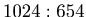is approximately equal to.

Graph 2.7Graph 2.8Example 2.6   Graph 2.9 and Graph 2.10 are the graphs of the lists {, n = 1,2,..,1024} and {, n = 1,2,..,710}. Please compare these two graphs．They have the same shape, and hence these graphs seem to have Fractal behavior (self-similarity). Note thatis approximately equal to.

Graph 2.9Graph 2.10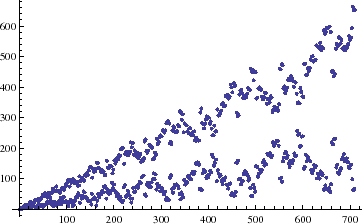Example 2.7   Graph 2.11 and Graph 2.12 are the graphs of the lists {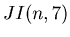, n = 1,2,..,1024} and {, n = 1,2,..,751}. Please compare these two graphs．They have the same shape, and hence these graphs seem to have Fractal behavior (self-similarity). Note thatis approximately equal to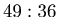.

Graph 2.11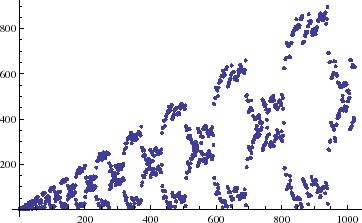Graph 2.12Example 2.8   Graph 2.13 and Graph 2.14 are the graphs of the lists {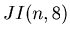, n = 1,2,..,1024} and {, n = 1,2,..,783}. Please compare these two graphs．They have the same shape, and hence these graphs seem to have Fractal behavior (self-similarity). Note that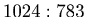is approximately equal to.

Graph 2.13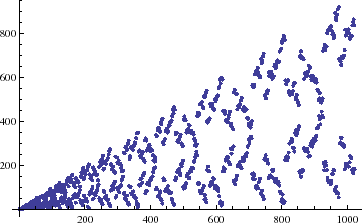Graph 2.14Example 2.9   Graph 2.15 and Graph 2.16 are the graphs of the lists {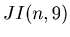, n = 1,2,..,1024} and {, n = 1,2,..,808}. Please compare these two graphs．They have the same shape, and hence these graphs seem to have Fractal behavior (self-similarity). Note that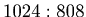is approximately equal to.

Graph 2.15Graph 2.16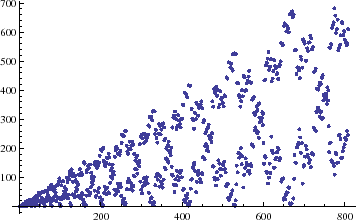Example 2.10   Graph 2.17 and Graph 2.18 are the graphs of the lists {, n = 1,2,..,1024} and {, n = 1,2,..,828}. Please compare these two graphs．They have the same shape, and hence these graphs seem to have Fractal behavior (self-similarity). Note thatis approximately equal to.

Graph 2.17Graph 2.18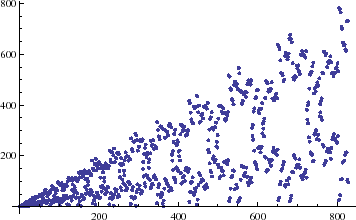From Example 2.2, ..., Example 2.10 the authors can get the following prediction.

Prediction 2.1   The graph of the list {, n = 1,2,..,} has the self-similarity with the ratio of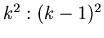.: Linear Josephus Problem. : The Self-Similarity of the Josephus Problems. : Introduction and the traditional Josephus Problem.